Anúncio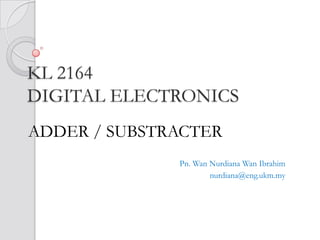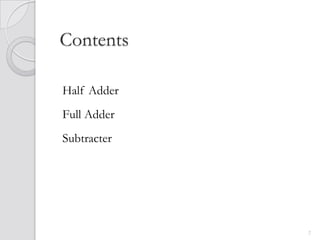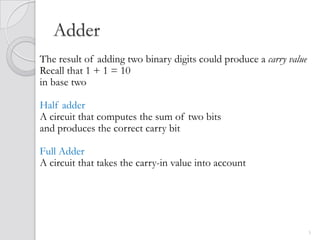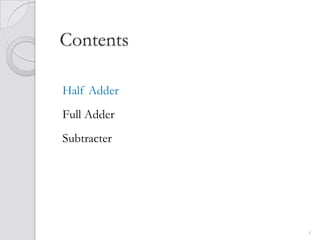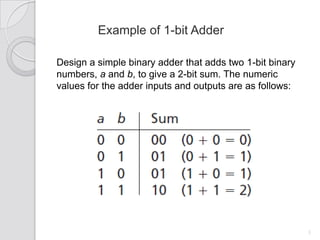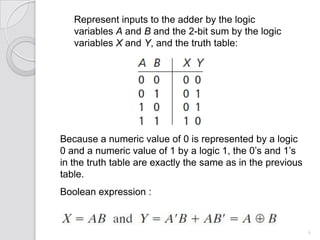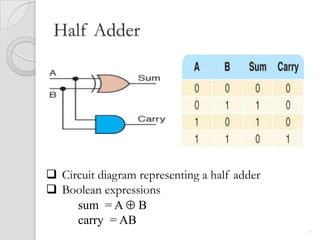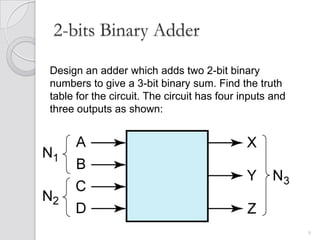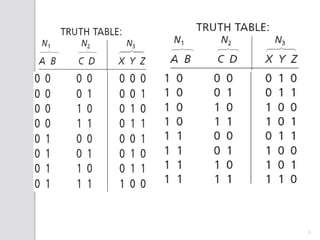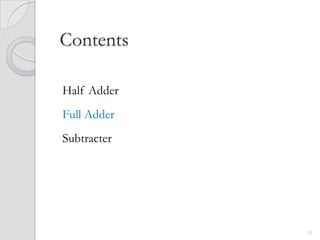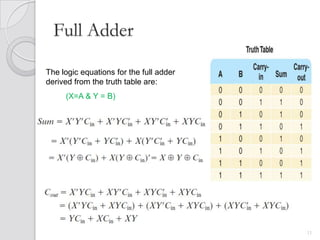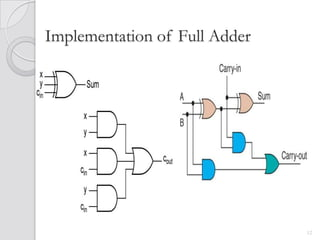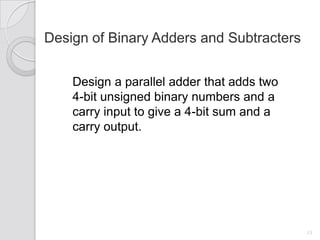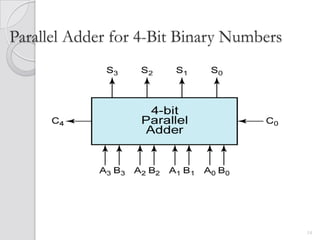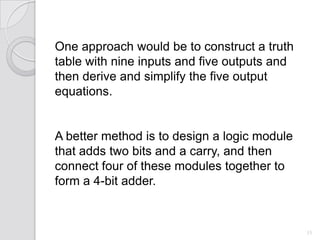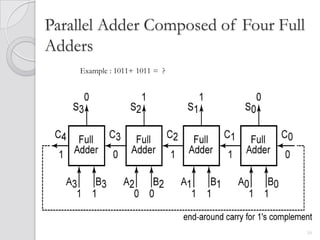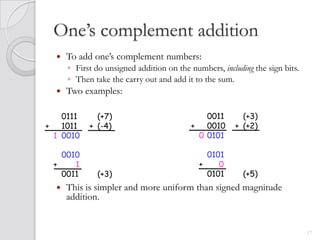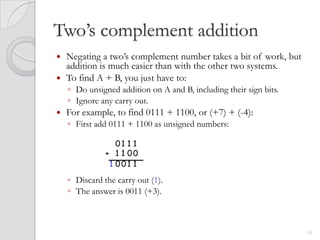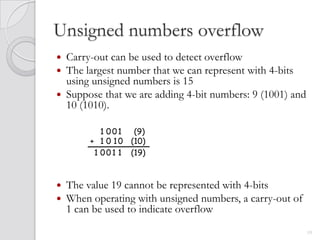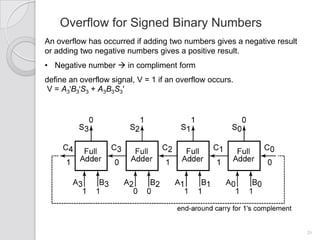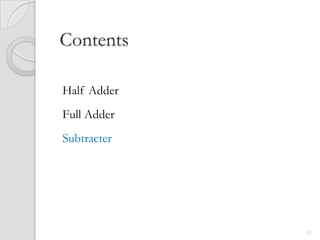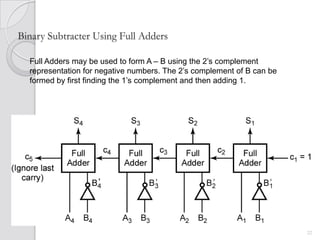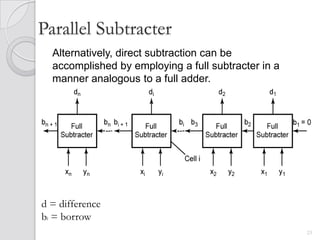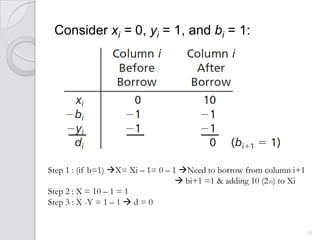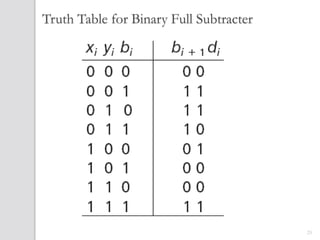1 de 25
Anúncio

1. KL 2164 DIGITAL ELECTRONICS ADDER / SUBSTRACTER Pn. Wan Nurdiana Wan Ibrahim nurdiana@eng.ukm.my
3. Adder The result of adding two binary digits could produce a carry value Recall that 1 + 1 = 10 in base two Half adder A circuit that computes the sum of two bits and produces the correct carry bit Full Adder A circuit that takes the carry-in value into account 3
5. Example of 1-bit Adder Design a simple binary adder that adds two 1-bit binary numbers, a and b, to give a 2-bit sum. The numeric values for the adder inputs and outputs are as follows: 5
6. Represent inputs to the adder by the logic variables A and B and the 2-bit sum by the logic variables X and Y, and the truth table: Because a numeric value of 0 is represented by a logic 0 and a numeric value of 1 by a logic 1, the 0’s and 1’s in the truth table are exactly the same as in the previous table. Boolean expression : 6
7. Half Adder  Circuit diagram representing a half adder  Boolean expressions sum = A B carry = AB 7
8. 2-bits Binary Adder Design an adder which adds two 2-bit binary numbers to give a 3-bit binary sum. Find the truth table for the circuit. The circuit has four inputs and three outputs as shown: 8
9. 9
11. Full Adder The logic equations for the full adder derived from the truth table are: (X=A & Y = B) 11
12. Implementation of Full Adder 12
13. Design of Binary Adders and Subtracters Design a parallel adder that adds two 4-bit unsigned binary numbers and a carry input to give a 4-bit sum and a carry output. 13
14. Parallel Adder for 4-Bit Binary Numbers 14
15. One approach would be to construct a truth table with nine inputs and five outputs and then derive and simplify the five output equations. A better method is to design a logic module that adds two bits and a carry, and then connect four of these modules together to form a 4-bit adder. 15
16. Parallel Adder Composed of Four Full Adders Example : 1011+ 1011 = ? 16
17. One’s complement addition  To add one’s complement numbers: ◦ First do unsigned addition on the numbers, including the sign bits. ◦ Then take the carry out and add it to the sum.  Two examples: 0111 (+7) 0011 (+3) + 1011 + (-4) + 0010 + (+2) 1 0010 0 0101 0010 0101 + 1 + 0 0011 (+3) 0101 (+5)  This is simpler and more uniform than signed magnitude addition. 17
18. Two’s complement addition  Negating a two’s complement number takes a bit of work, but addition is much easier than with the other two systems.  To find A + B, you just have to: ◦ Do unsigned addition on A and B, including their sign bits. ◦ Ignore any carry out.  For example, to find 0111 + 1100, or (+7) + (-4): ◦ First add 0111 + 1100 as unsigned numbers: 01 1 1 + 1 1 00 1 001 1 ◦ Discard the carry out (1). ◦ The answer is 0011 (+3). 18
19. Unsigned numbers overflow  Carry-out can be used to detect overflow  The largest number that we can represent with 4-bits using unsigned numbers is 15  Suppose that we are adding 4-bit numbers: 9 (1001) and 10 (1010). 1 001 (9) + 1 0 10 (10) 1 001 1 (19)  The value 19 cannot be represented with 4-bits  When operating with unsigned numbers, a carry-out of 1 can be used to indicate overflow 19
20. Overflow for Signed Binary Numbers An overflow has occurred if adding two numbers gives a negative result or adding two negative numbers gives a positive result. • Negative number  in compliment form define an overflow signal, V = 1 if an overflow occurs. V = A3′B3′S3 + A3B3S3′ 20